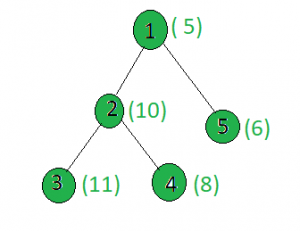# Count the nodes in the given tree whose weight is even

Given a tree, and the weights of all the nodes, the task is to count the number of nodes whose weight is even.

Examples:

Input:Output: 3
Only the weights of the nodes 2, 4 and 5 are even.

## Recommended: Please try your approach on {IDE} first, before moving on to the solution.

Approach: Perform dfs on the tree and for every node, check if it’s weight is divisible by 2 or not. If yes then increment count.

Below is the implementation of the above approach:

## C++

 `// C++ implementation of the approach ` `#include ` `using` `namespace` `std; ` ` `  `int` `ans = 0; ` ` `  `vector<``int``> graph; ` `vector<``int``> weight(100); ` ` `  `// Function to perform dfs ` `void` `dfs(``int` `node, ``int` `parent) ` `{ ` `    ``// If weight of the current node is even ` `    ``if` `(weight[node] % 2 == 0) ` `        ``ans += 1; ` ` `  `    ``for` `(``int` `to : graph[node]) { ` `        ``if` `(to == parent) ` `            ``continue``; ` `        ``dfs(to, node); ` `    ``} ` `} ` ` `  `// Driver code ` `int` `main() ` `{ ` `    ``int` `x = 15; ` ` `  `    ``// Weights of the node ` `    ``weight = 5; ` `    ``weight = 10; ` `    ``weight = 11; ` `    ``weight = 8; ` `    ``weight = 6; ` ` `  `    ``// Edges of the tree ` `    ``graph.push_back(2); ` `    ``graph.push_back(3); ` `    ``graph.push_back(4); ` `    ``graph.push_back(5); ` ` `  `    ``dfs(1, 1); ` ` `  `    ``cout << ans; ` ` `  `    ``return` `0; ` `} `

## Java

 `// Java implementation of the approach  ` `import` `java.util.*;  ` ` `  `class` `GFG  ` `{  ` `    ``static` `int` `ans = ``0``;  ` `     `  `    ``@SuppressWarnings``(``"unchecked"``)  ` `    ``static` `Vector[] graph = ``new` `Vector[``100``];  ` `    ``static` `int``[] weight = ``new` `int``[``100``]; ` `     `  `    ``// Function to perform dfs  ` `    ``static` `void` `dfs(``int` `node, ``int` `parent)  ` `    ``{  ` `        ``// If weight of the current node is even  ` `        ``if` `(weight[node] % ``2` `== ``0``)  ` `            ``ans += ``1``;  ` `     `  `        ``for` `(``int` `to : graph[node])  ` `        ``{  ` `            ``if` `(to == parent)  ` `                ``continue``;  ` `            ``dfs(to, node);  ` `        ``}  ` `    ``}  ` `     `  `    ``// Driver code  ` `    ``public` `static` `void` `main(String[] args) ` `    ``{  ` `        ``int` `x = ``15``;  ` `     `  `    ``for` `(``int` `i = ``0``; i < ``100``; i++)  ` `            ``graph[i] = ``new` `Vector<>();  ` `         `  `        ``// Weights of the node  ` `        ``weight[``1``] = ``5``;  ` `        ``weight[``2``] = ``10``;  ` `        ``weight[``3``] = ``11``;  ` `        ``weight[``4``] = ``8``;  ` `        ``weight[``5``] = ``6``;  ` `     `  `        ``// Edges of the tree  ` `        ``graph[``1``].add(``2``);  ` `        ``graph[``2``].add(``3``);  ` `        ``graph[``2``].add(``4``);  ` `        ``graph[``1``].add(``5``);  ` `     `  `        ``dfs(``1``, ``1``);  ` `     `  `        ``System.out.println(ans); ` `    ``}  ` `} ` ` `  `// This code is contributed by shubhamsingh10 `

## Python3

 `# Python3 implementation of the approach ` `ans ``=` `0` ` `  `graph ``=` `[[] ``for` `i ``in` `range``(``100``)] ` `weight ``=` `[``0``] ``*` `100` ` `  `# Function to perform dfs ` `def` `dfs(node, parent): ` `    ``global` `ans ` `     `  `    ``# If weight of the current node is even ` `    ``if` `(weight[node] ``%` `2` `=``=` `0``): ` `        ``ans ``+``=` `1` `     `  `    ``for` `to ``in` `graph[node]: ` `        ``if` `(to ``=``=` `parent): ` `            ``continue` `        ``dfs(to, node) ` ` `  `# Driver code ` `x ``=` `15` ` `  `# Weights of the node ` `weight[``1``] ``=` `5` `weight[``2``] ``=` `10` `weight[``3``] ``=` `11` `weight[``4``] ``=` `8` `weight[``5``] ``=` `6` ` `  `# Edges of the tree ` `graph[``1``].append(``2``) ` `graph[``2``].append(``3``) ` `graph[``2``].append(``4``) ` `graph[``1``].append(``5``) ` ` `  `dfs(``1``, ``1``) ` `print``(ans) ` ` `  `# This code is contributed by SHUBHAMSINGH10 `

## C#

 `// C# implementation of the approach  ` `using` `System; ` `using` `System.Collections.Generic; ` ` `  `class` `GFG  ` `{  ` `    ``static` `int` `ans = 0;  ` `    ``static` `List<``int``>[] graph = ``new` `List<``int``>;  ` `    ``static` `int``[] weight = ``new` `int``; ` `      `  `    ``// Function to perform dfs  ` `    ``static` `void` `dfs(``int` `node, ``int` `parent)  ` `    ``{  ` `        ``// If weight of the current node is even  ` `        ``if` `(weight[node] % 2 == 0)  ` `            ``ans += 1;  ` `      `  `        ``foreach` `(``int` `to ``in` `graph[node])  ` `        ``{  ` `            ``if` `(to == parent)  ` `                ``continue``;  ` `            ``dfs(to, node);  ` `        ``}  ` `    ``}  ` `      `  `    ``// Driver code  ` `    ``public` `static` `void` `Main(String[] args) ` `    ``{       ` `        ``for` `(``int` `i = 0; i < 100; i++)  ` `            ``graph[i] = ``new` `List<``int``>();  ` `          `  `        ``// Weights of the node  ` `        ``weight = 5;  ` `        ``weight = 10;  ` `        ``weight = 11;  ` `        ``weight = 8;  ` `        ``weight = 6;  ` `      `  `        ``// Edges of the tree  ` `        ``graph.Add(2);  ` `        ``graph.Add(3);  ` `        ``graph.Add(4);  ` `        ``graph.Add(5);  ` `      `  `        ``dfs(1, 1);  ` `      `  `        ``Console.WriteLine(ans); ` `    ``}  ` `} ` ` `  `// This code is contributed by Rajput-Ji `

Output:

```3
```

Attention reader! Don’t stop learning now. Get hold of all the important DSA concepts with the DSA Self Paced Course at a student-friendly price and become industry ready.

My Personal Notes arrow_drop_upCheck out this Author's contributed articles.

If you like GeeksforGeeks and would like to contribute, you can also write an article using contribute.geeksforgeeks.org or mail your article to contribute@geeksforgeeks.org. See your article appearing on the GeeksforGeeks main page and help other Geeks.

Please Improve this article if you find anything incorrect by clicking on the "Improve Article" button below.

Improved By : SHUBHAMSINGH10, Rajput-Ji

Article Tags :
Practice Tags :

Be the First to upvote.

Please write to us at contribute@geeksforgeeks.org to report any issue with the above content.